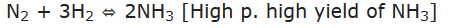It states that a change in any of the factors that determine the equilibrium conditions of a system will cause the system to change in such a manner so as to reduce or to counteract the effect of the change. This is applicable to all physical and chemical equilibria.

There are three main factors which affect the state of equilibrium. They are:

• Concentration
• Temperature
• Pressure

## Effect of Change of Concentration

When the concentration of any of the reactants or products in a reaction at equilibrium is changed, the composition of the equilibrium changes so as to minimize the effect.

## Effect of Change in Pressure

No effect of pressure on equilibria having same moles of reactants and products.

E.g., N2 + O2 ↔ 2NO

When there is change in the number of moles, the equilibrium will shift in the direction having smaller number of moles when the pressure is increased and vice-versa, e.g.,## Effect of Inert Gas Addition

If the volume is kept constant there is no effect on equilibrium after the addition of an inert gas.
Reason: This is because the addition of an inert gas at constant volume does not change the partial pressure or the molar concentration.
The reaction quotient changes only if the added gas is involved in the reaction.

## Effect of Temperature Change

When the temperature of the system is changed (increased or decreased), the equilibrium shifts in opposite direction in order to neutralize the effect of change. In exothermic reaction low temperature favors forward reaction e.g.,but practically very low temperature slows down the reaction and thus a catalyst is used. In case of endothermic reaction, the increase in temperature will shift the equilibrium in the direction of the endothermic reaction.

## Effect of a Catalyst

Catalyst has no effect on the equilibrium composition of a reaction mixture.
Reason: Since catalyst increases the speed of both the forward and backward reactions to the same extent in a reversible reaction.

Click on a star to rate it!

Average rating 0 / 5. Vote count: 0

No votes so far! Be the first to rate this post.

As you found this post useful...